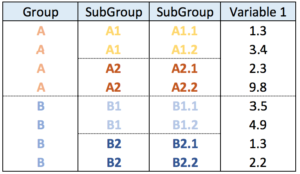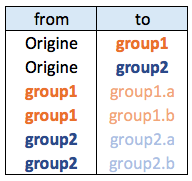# Zoomable circle packing with R and circlePacker

The circlePacker package allows to build interactive and zoomable circle packing charts. This post explains how to use the package with different kind of input datasets.

The `circlepackeR` package allows to build interactive circle packing. Click on a group, and a smooth zoom will reveal the subgroups behind it.

Circle packing is a visualization method for hierarchical data. This kind of data can be stored in 2 main ways:

• Nested data frame
• Edge listNested data frameEdge list

# Circular packing fom nested data frame

In a nested data frame, each line represents a leaf of the organization. Each column represents a level of the organization.

This data format will require the `data.tree` library to reformat the input dataset into something readable by `circlepackeR`.

``````# Circlepacker package
library(circlepackeR)
# devtools::install_github("jeromefroe/circlepackeR") # If needed

# create a nested data frame giving the info of a nested dataset:
data <- data.frame(
root=rep("root", 15),
group=c(rep("group A",5), rep("group B",5), rep("group C",5)),
subgroup= rep(letters[1:5], each=3),
subsubgroup=rep(letters[1:3], 5),
value=sample(seq(1:15), 15)
)

# Change the format. This use the data.tree library. This library needs a column that looks like root/group/subgroup/..., so I build it
library(data.tree)
data\$pathString <- paste("world", data\$group, data\$subgroup, data\$subsubgroup, sep = "/")
population <- as.Node(data)

# Make the plot
#circlepackeR(population, size = "value")

# You can custom the minimum and maximum value of the color range.
p <- circlepackeR(population, size = "value", color_min = "hsl(56,80%,80%)", color_max = "hsl(341,30%,40%)")

# save the widget
# library(htmlwidgets)
# saveWidget(p, file=paste0( getwd(), "/HtmlWidget/circular_packing_circlepackeR2.html"))``````

# Circular packing fom edge list

The edge list format has at least 2 columns. It describes all the edges of the data.

This format is widely spread. In this example, we just convert it to a nested data frame before plotting it as seen above.

``````# Circlepacker package
library(circlepackeR)
# devtools::install_github("jeromefroe/circlepackeR") # If needed

# Let's use the 'flare dataset' (stored in the ggraph library)
library(ggraph)
data_edge <- flare\$edges
data_edge\$from <- gsub(".*\\.","",data_edge\$from)
data_edge\$to <- gsub(".*\\.","",data_edge\$to)
head(data_edge)   # This is an edge list

# We need to convert it to a nested data frame. the data.tree library is our best friend for that:
library(data.tree)
data_tree <- FromDataFrameNetwork(data_edge)
data_nested <- ToDataFrameTree(data_tree,
level1 = function(x) x\$path,
level2 = function(x) x\$path,
level3 = function(x) x\$path,
level4 = function(x) x\$path)[-1,-1]
data_nested <- na.omit(data_nested)

# Now we can plot it as seen before!
data_nested\$pathString <- paste("roots", data_nested\$level1, data_nested\$level2, data_nested\$level3, data_nested\$level4, sep = "/")
data_nested\$value=1
data_Node <- as.Node(data_nested)
p <- circlepackeR(data_Node, size = "value")
#p

# save the widget
# library(htmlwidgets)
# saveWidget(p, file=paste0( getwd(), "/HtmlWidget/circular_packing_circlepackeR1.html"))``````

Related chart types

## Contact

This document is a work by Yan Holtz. Any feedback is highly encouraged. You can fill an issue on Github, drop me a message on Twitter, or send an email pasting yan.holtz.data with gmail.com.